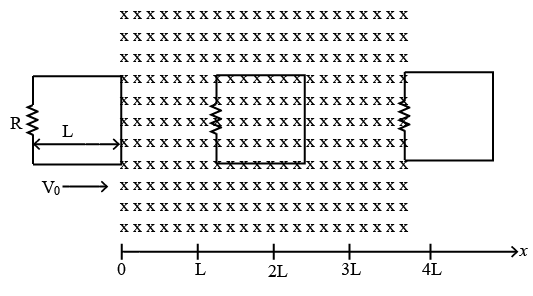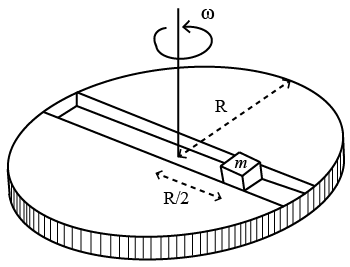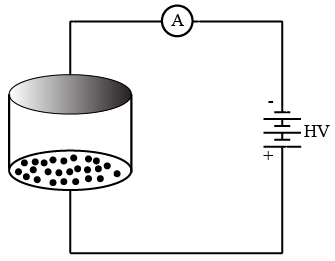Instructions

For the following questions answer them individually

Question 11

Question 12

Question 13

Question 14

# A rigid wire loop of square shape having side of length L and resistance R is moving along the x-axis with a constant velocity $$v_0$$ in the plane of the paper. At t = 0, the right edge of the loop enters a region of length 3L where there is a uniform magnetic field $$B_0$$, into the plane of the paper, as shown in the figure. For sufficiently large $$v_0$$, the loop eventually crosses the region. Let x be the location of the right edge of the loop. Let v(x), I(x) and F(x) represent the velocity of the loop, current in the loop, and force on the loop, respectively, as a function of x. Counter-clockwise current is taken as positive.Which of the following schematic plot(s) is(are) correct? (Ignore gravity)

Instructions

PARAGRAPH 1

A frame of reference that is accelerated with respect to an inertial frameof reference is called a non-inertial frame of reference. A coordinate system fixed on a circular disc rotating about a fixed axis with a constant angular velocity $$\omega$$ is an example of a non-inertial frame of reference. The relationship between the force $$\overrightarrow{F}_{rot}$$ experienced by a particle of mass m moving on the $$\overrightarrow{F}_{in}$$ experienced by the particle in an inertial frame of reference is
$$\overrightarrow{F}_{rot} = \overrightarrow{F}_{in} + 2m(\overrightarrow{V}_{rot} \times \overrightarrow{\omega}) + m(\overrightarrow{\omega} \times \overrightarrow{r}) \times \overrightarrow{\omega}$$,
where $$\overrightarrow{v}_{rot}$$ is the velocity of the particle in the rotating frameof reference and $$\overrightarrow{r}$$. is the position vector of the particle with respect to the centre of the disc.
Now consider a smooth slot along a diameter of a disc of radius R rotating counter-clockwise with a constant angular speed $$\omega$$ about its vertical axis through its center. We assign a
coordinate system with the origin at the center of the disc, the x-axis along the slot, the y-axis perpendicular to the slot and the z-axis along the rotation axis $$(\overrightarrow{\omega} = \omega \hat{k})$$. A small block of mass m is gently placed in the slot at $$\overrightarrow{r} = \left(\frac{R}{2}\right)\hat{i}$$ at t = 0 and is constrained to move only along the slot.Question 15

Question 16

# The net reaction of the disc on the block is

Instructions

PARAGRAPH 2

Consider an evacuated cylindrical chamber of height h having rigid conducting plates at the ends and an insulating curved surface as shownin the figure. A number of spherical balls made
of a light weight and soft material and coated with a conducting material are placed on the bottom plate. The balls have a radius r<<h. Nowa high voltage source (HV) is connected across
the conducting plates such that the bottom plate is at $$+ V_0$$ and the top plate at $$- V_0$$. Due to their conducting surface, the balls will get charged, will become equipotential with the plate and are repelled by it. The balls will eventually collide with the top plate, where the coefficient of restitution can be taken to be zero due to the soft nature of the material of the balls. The electric field in the chamber can be considered to be that of a parallel plate capacitor. Assume that there are no collisions between the balls and the interaction between them is negligible. (Ignore gravity)Question 17

Question 18

# The average current in the steady state registered by the ammeter in the circuit will be

Instructions

For the following questions answer them individually

Question 19

Question 20

OR